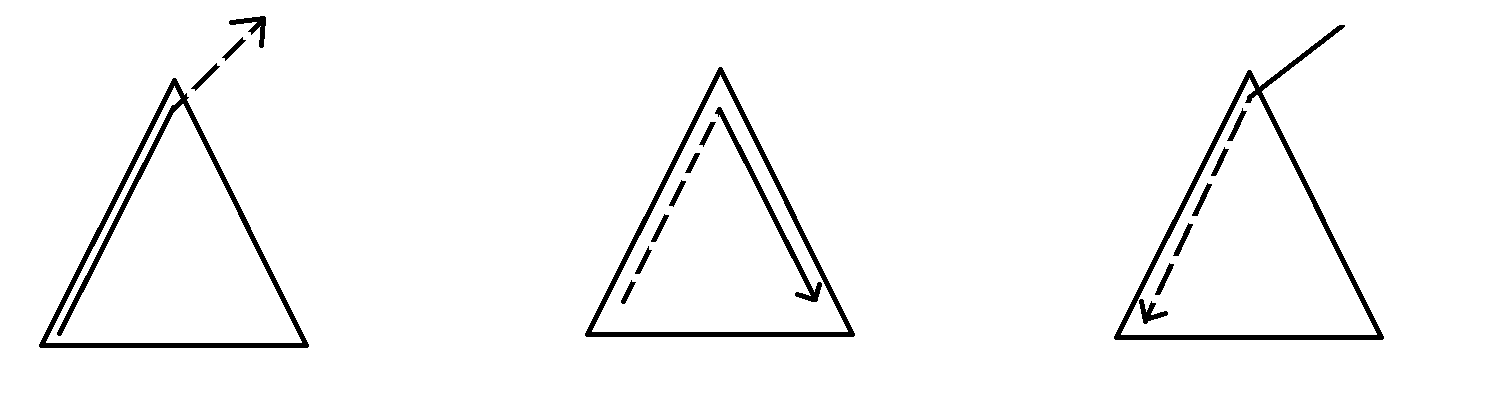zc
2019-12-21 19:47:00

lv,rv:左右端点的值
s: 区间答案
l:以左端开始最长下降
r:以右端结束的最长上升
L:以左端开始最长先上升(再下降)
R:以右端结束最长先上升(再下降)zc
2019-12-21 19:47:00zc
2019-12-21 19:47:00zc
2019-12-21 19:47:00

1. 买,第二天卖了

2. 不买(相当于买了然后卖了)

3. 买了,过几天再卖(相当于买了了,接下来卖了再买了)

d-1次背包即可zc
2019-12-21 19:47:00zc
2019-12-21 19:47:00

(tips:getline,ssstream)zc
2019-12-21 19:47:00

1.如果全是0

2.没有0

3.没有负数

4.没有正数zc
2019-12-21 19:47:001. x子树内\rightarrow x \rightarrow x子树外(包括x)

2. x子树内(包括x)\rightarrow x \rightarrow x子树内

3. x子树外\rightarrow x \rightarrow x子树内

1. ans += V_x \times (f_x-g_x) \times (n-siz_x+1)(可以在x的子树中随机选一个往子树外面走(可以指走到x))

2. ans += V_x \times (g_{to}-f_{to}) \times (siz_x-siz_{to}-1)

ans+= V_x \times (siz_x-1)(可以从x直接向下走)

3. 我们需要记录x子树外与x的距离为偶数和与x的距离为奇数的点的个数,分别记作uf_x,ug_x

我们可以通过bfs从上到下来处理,那么

uf_x=ug_{fa_x}+g_{fa_x}-f_x

ug_x=uf_{fa_x}+f_{fa_x}-g_x

ans += V_x \times (uf_x-ug_x) \times siz_xzc
2019-12-21 19:47:00zc
2019-12-21 19:47:00

f[i][j]表示在i的子树中与i距离为j的点数

g[i][j]表示以i为根的子树中,点对(x,y)满足x,ylca(x,y)距离都是d,并且lca(x,y)i的距离为d-j的点对数

g[i][j] = \sum g[to][j+1]

ans = \sum_{i=1}^n (g[i]+g[i][j] \times f[to][j-1])

n \le 100000

Search
search